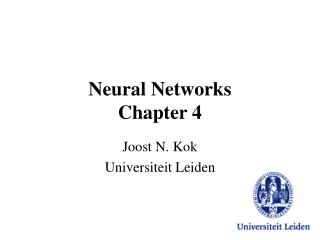DownloadDownload PresentationNeural Networks Chapter 4

# Neural Networks Chapter 4

Télécharger la présentation## Neural Networks Chapter 4

- - - - - - - - - - - - - - - - - - - - - - - - - - - E N D - - - - - - - - - - - - - - - - - - - - - - - - - - -
##### Presentation Transcript

1. Neural NetworksChapter 4 Joost N. Kok Universiteit Leiden

2. Hopfield Networks • Optimization Problems (like Traveling Salesman) can be encoded into Hopfield Networks • Fitness corresponds to energy of network • Good solutions are stable points of the network

3. Hopfield Networks • Three Problems • Weighted Matching • Traveling Salesman • Graph Bipartitioning

4. Hopfield Networks • Weighted matching Problem: • Let be given N points with distances dij • Connect points together in pairs such that the total sum of distances is as small as possible

5. Hopfield Networks • Variables: nij (i<j) with values 0/1 • Constraint: Sj nij = 1 for all i • Optimize:Si<j dij nij

6. Hopfield Networks • Penalty Term approach: put constraints in optimization criterion • Weights and thresholds of Hopfield Network can be derived from

7. Hopfield Networks • Travelling Salesman Problem (TSP):Given N cities with distances dij .What is the shortest tour?

8. Hopfield Networks • Construct a Hopfield network with N2 nodes • Semantics: nia = 1 iff town i on position a in tour

9. Hopfield Networks • Constraints:

10. Hopfield Networks • 0/1 Nodes • Nodes within each row connected with weight –g • Nodes within each column connected with weight –g • Each node is connected to nodes in columns left and right with weight –dij • (Often) continuousactivation

11. Hopfield Networks

12. Hopfield Networks • Graph bipartitioning: divide nodes in two sets of equal size in such a way as to minimize the number of edges going between the sets • +1/-1 Nodes • 0/1 Connection matrix Cij

13. Hopfield Networks

14. Hopfield Networks